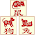## Reverse Words in a String

Given an input string, reverse the string word by word.
For example,
Given s = "the sky is blue",
return "blue is sky the".
Clarification:
• What constitutes a word?
A sequence of non-space characters constitutes a word.
• Could the input string contain leading or trailing spaces?
Yes. However, your reversed string should not contain leading or trailing spaces.
• How about multiple spaces between two words?
Reduce them to a single space in the reversed string.

### Analysis:

The clarification is very important, during the interview, one should think of these points without hint, and then implement the algorithm.

By checking the clarifications, the programming is straightforward, here provides a simple version which uses buffer strings:
(1)Loop from start to the end of the string:
(a) if current char is space and word string is empty, continue.
(b) if current char is space but word string is NOT empty, which means we meet the end of word, then output the word, reset the word, continue.
(c) if current char is non-space char, add this char to the word string, continue
(2)Handle the last word:
(a) if the last word is empty, which means the input is empty, or the input has only spaces, or the last char/chars are spaces.  Then just remove the last space in the output string and return.
(b) if the last word is not empty, add the last word to the front of the output string, then remove the last space in the output string and return.

### Code (C++):

class Solution {
public:
void reverseWords(string &s) {
string word; //tmp string to store each word
string res; // result string
int i=0;
while (i<s.size()){
if (char(s[i])==' ' && word.empty()){i++;continue;} //multiple spaces
if (char(s[i])==' ' && !word.empty()){ //first space after a word
res = word+" "+ res; //store the word
word=""; //reset the word
i++; continue;
}
if (char(s[i])!=' '){word=word+char(s[i]);i++; continue;} //non-space chars
}

if (!word.empty()){ //last word
s = word+" "+res;
}else{
s = res;
}
s = s.substr(0,s.size()-1); //eliminate the last space
}
};


### Code (Python):

class Solution:
# @param s, a string
# @return a string
def reverseWords(self, s):
res = ""    # result string
word = ""   # single word string
for ch in s:
if (ch!=' '):
word+=ch
if (ch==' '):
if (len(word)!=0):
if (res!=""):   # add space between words
res = ' ' + res
res = word + res
word = ""

if (len(word)!=0):  #handle the final word
if (res!=""):
res = ' ' + res
res = word + res

return res



1.Good work.
Your Python solution is too 'C++'. Here is my Python solution.

class Solution:
# @param s, a string
# @return a string
def reverseWords(self, s):
return ' '.join(s,split(' ')[-1::-1])

1.Submission Result: Wrong Answer
Input: " "
Output: " "
Expected: ""

2.This comment has been removed by the author.

2.Can you assume there is only one space between words?

3.Really nice code. Helped me a lot since there are not many c++ oriented solutions for such problems out there. Thank you.

4.' '.join(s.split(" ")[::-1])